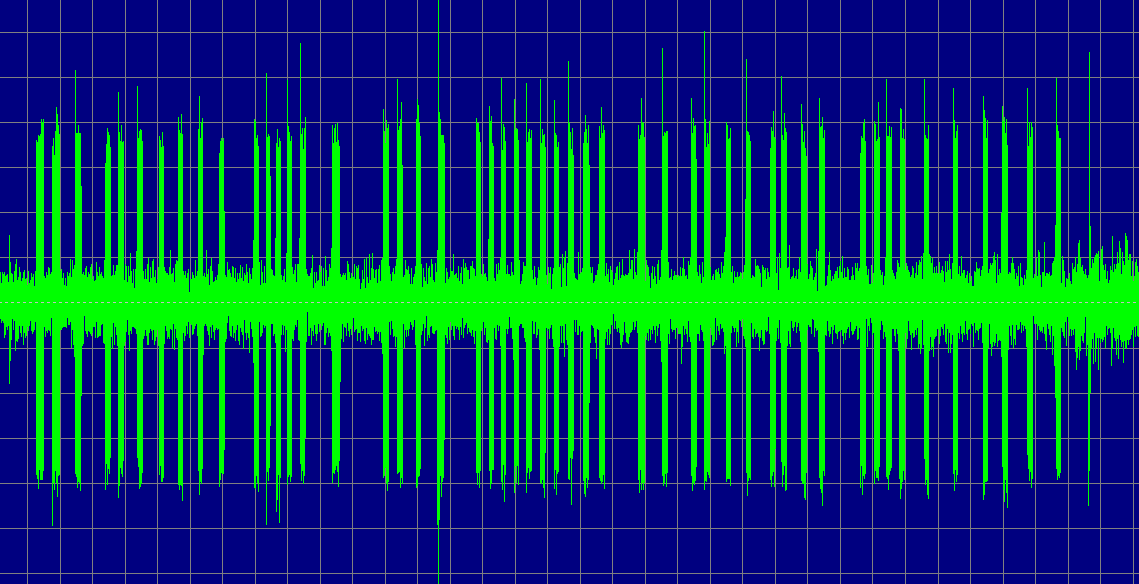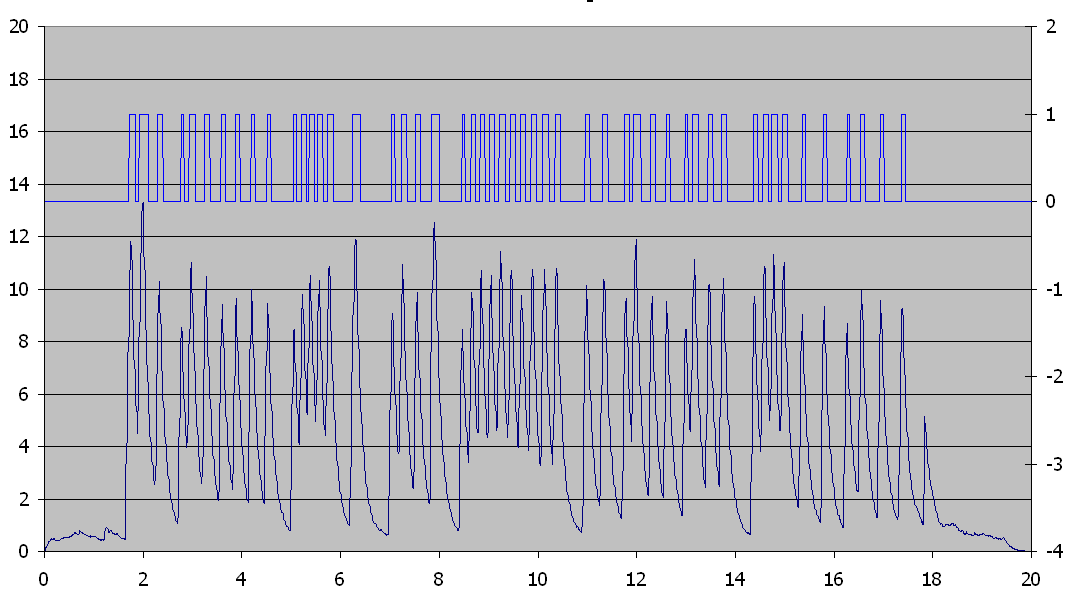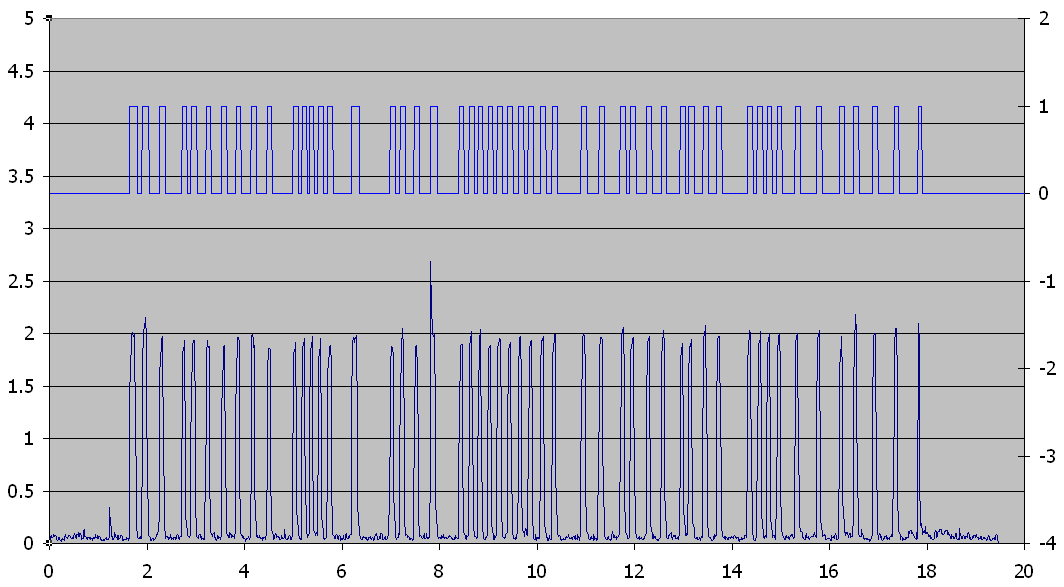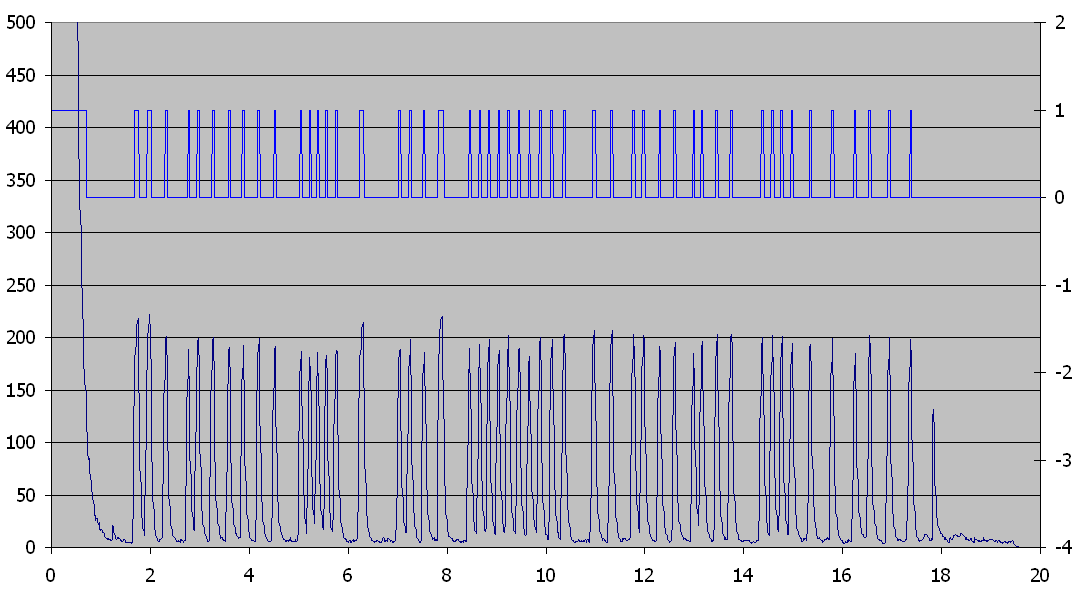BBC Homepage

# BBC-based Concurrent Code Audio Testbed

The following data was generated by holding a telephone handset against a PC microphone and hitting the "8" key many times (50, to be exact). It was captured using the GoldWave software package and stored in an 8-bit monaural WAV file at a sampling rate of 8 kHz. Examination of the data shows that most of the tones lasted approximately 100 ms. At the very end of the data is a very narrow spike that was the result of a door opening.The next step was to analyze the captured data using radiometric techniques (i.e., energy detection as opposed to coherent demodulation or other techniques that rely on particular characteristics of the source signal). The following difference equation was used:

vout[n] = decay*vout[n-1] + (vin[n])2/dt

This resulting exponentially decaying impulse response produces an output that is a moving average of the energy content of the signal. The smaller the decay factor (which must be less than unity for stability) the shorter the time period over which the average is taken.

For simplicity sake, a value of 0.999 was chosen for the decay without any particular concern for whether it was a reasonable value (the code was being tested at this point, not the algorithm) and that produced very noticeable spikes corresponding to each sound pulse. Based on the plot, a discriminator threshold of (8.0, 6.0) was chosen where the first value is threshold for the discrimator to transition from LO to HI and the second is the threshold for it to transition from HI to LO. The resulting hysteresis provides debouncing similar to a Schmidt trigger.

The following shows the output of the radiometer (bottom trace, left axis) and the discriminator (top trace, right axis). Notice that there are exactly 50 output pulses from the discriminator with a clear time correlation to the original sound pulses. Also notice that the transient due to the door is suppressed well below the other pulses. However, the radiometer decay setting is too high since the output should decay to close to the background level between sound pulses. As a result, the discriminator performance is very sensitive to the selection of threshold limits.Noting that telephone DTMF signals are all above 600 Hz (about 13 samples at a sampling rate of 8 kHz), we want the radiometer to average over at least that long to avoid intra-symbol fluctuation. Our nominal symbol duration of 100 ms corresponds to 800 samples and we would like the radiometer output to rise and decay within a fraction of that time, say perhaps 100 samples. By adjusting the radiometer decay setting to 0.9, the following output was obtained. The threshold limits were set to (1.0, 0.1). While this resulted in very clean and well separated pulses of nearly uniform height, thus making the discrimator thresholds very tolerant, notice that the pulse due to the door opening is captured as well.To make a more reasoned selection for the decay setting, note that the input from N samples prior is only weighted (decay)N as much as the present input. If we want this sample to be weight one-half as much as the present sample, then we set the decay ratio to the Nth-root of 0.5. For N=100, this results in a decay ratio of 0.993 and the following output, which appears to be a quite reasonable choice. The pulses are nearly the same amplitude and, although the discriminator thresholds chosen (2.0,1.0) still picked up the noise from the door, they clearly could have been chosen to discriminate against it.In order to tie the control parameters more closely to physical concepts, the following conversions were made:

First, an IIR filter that computes a moving average over a fairly wide time window was used to determine the approximate DC value for each sample. The user is asked for the width of this window, in seconds, and the filter coefficients are computed based on the following relation:

avg[n] = a*avg[n-1] + b*v_in[n]

Roughly speaking, if the window corresponds to N = window*SamplesPerSecond samples, then only 1/N of the current input value should be included in the computation of the average.

Furthermore, if the signal is truly a DC value, then

avg[n] = avg[n-1] = v_in[n]

Therefore

a = 1 - b = 1/N

where

N = window*dt

and dt is the time increment between samples.

Next, the User is asked for a relaxation time for the radiometer. The relaxation time is roughly the time that it takes the output to return to the quiescent level after an energy pulse ends. This is converted into a decay coefficient:

decay_coefficient = exp(ln(0.5)*dt/recovery_time)

Finally, the User is asked for a nominal threshold value for the discriminator and then for the hysteresis width as a fraction of the threshold value. These are converted to the actual threshold values as follows:

vlh = vth*(1 + hystesis/2 )

vhl = vth*(1 - hystesis/2)

In order to provide some normalization for the discriminator threshold values, the radiometer data is divided by the relaxation time. This is because the radiometer is essentially integrating the signal over a period of time proportional to the relaxation time and, hence, its output is also proportion to this parameter.

With these modifications, the following is the result for the following User inputs:

• Time Window: 0.25 seconds

• Relaxation Time: 25 ms

• Threshold: 150

• Hysteresis: 10%

Notice the initial output spike while the radiometer's averaging filter settles. The initial discrimator output pulse can be easily suppressed by simply forcing it LO until the radiometer output first falls below the detection threshold.